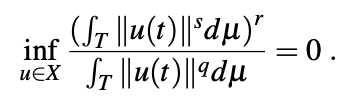# Further applications of two minimax theorems

• D. Giandinoto Stockholm University, Sweden

### Abstract

In this paper, we deal with new applications of two minimax theorems of B. Ricceri (,). Here is a particular case of one of the results that we obtain: Let (T,F,μ) be a non-atomic measure space, with μ(T) < +∞, (E , ∥ · ∥) a real Banach space, I ⊆ E an unbounded set whose closure does not contain 0. Moreover, let p, q, r, s be four numbers such that 0 < s < q ≤ p, p ≥ 1, r > 1. Set X := {f ∈ Lp(T,E) : f(T) ⊆ I}. Then, one hasPublished
2023-02-06
Issue
Section
Articoli